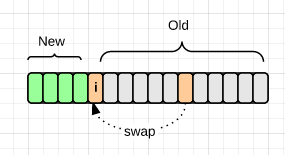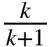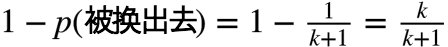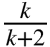# 几个常见的概率问题

## 1. 洗牌function shuffle(array) {
var m = array.length, i;

// While there remain elements to shuffle…
// 为了方便这里是从后往前遍历的
while (m) {

// Pick a remaining element…
i = Math.floor(Math.random() * m--);

// And swap it with the current element.
var t = array[m];
array[m] = array[i];
array[i] = t;
}

}

## 2. 随机数生成器的转换

int Rand3()
{
int x = 5;
while(x >= 3)
x = Rand5();
return x;
}## 3. 蓄水池抽样

Init : a reservoir with the size： k
for i= k+1 to n
M=random(1, i);
if( M < k)
SWAP the Mth value and ith value
end for  

1. 当 n == k 时，每个元素出现在蓄水池的概率都是1，即2. 当 n == k+1 时，第一次考察发生在元素 k+1 处，根据算法，它被放入蓄水池的概率为；对原蓄水池中的任一元素，该次考察后被换出去的概率等于：所以，元素依然在蓄水池内的概率为3. 当 n == k+2 时，根据2，前 k+1 内的元素在第二次考察（即考察第 k+2 个元素）前在蓄水池内的概率为同样的，第 k+2 个元素被放入蓄水池的概率为对前 k+1 的元素，在第二次考察后在蓄水池要求 1)之前就在蓄水池；2)这次没有被交换出去：也符合条件。

4. 推广上述过程即可得证。